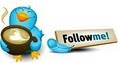#Use of two Earlier functions causes calculation to run forever• ### Question

•Number of rows in table: 1.8MM

Single earlier statement:

=calculate(
countrows(distinct(timesheet[proj_name]))
,all(timesheet)
,timesheet[week_end_date]=earlier(timesheet[week_end_date])

)

The above takes about 15 seconds to calculate

Double earlier statement:

=calculate(
countrows(distinct(timesheet[proj_name]))
,all(timesheet)
,timesheet[week_end_date]=earlier(timesheet[week_end_date])
,timesheet[resource_number]=earlier(timesheet[resource_number])

)

this should return for each week and each resource, the number of projects worked on

Never waited for it to finish calculating (over 90 minutes before I killed it).  For reference sake, the second formula (against 1.8MM records) should produce 342,872 distinct values.

Am I asking Powerpivot to do too much, or am I approching the solution in the wrong way?

Any thoughts?

Thanks,

Dave

Thursday, January 3, 2013 10:31 PM

•What I would suggest is to concatenate the columns and then just do one earlier function. Something like

1) Create a new calculated column called temp which concatenates timesheet[week_end_date] and timesheet[resource_number] and hide it.

=timesheet[week_end_date] + ',' + timesheet[resource_number]

2) Create the calculated measure now

`=calculate(    countrows(distinct(timesheet[proj_name]))    ,all(timesheet)    ,timesheet[temp]=earlier(timesheet[temp])            )`

This should get rid of the unnecessary iteration. I didnt check the syntax but I am guessing this should work.

Update

I was thinking about it and I thought a quicker and elegant way would be

`=CALCULATE(countrows(distinct(timesheet[proj_name])) , ALLEXCEPT( ‘timesheet’, timesheet[week_end_date], timesheet[resource_number] ) )`
Again, didnt check the syntax but you get the idea!

Cheers,
Jason | www.SqlJason.comFriday, January 4, 2013 3:08 PM
•The following calculated columns should return the expected result:

=CALCULATE(
DISTINCTCOUNT(timesheet[proj_name]))
,ALLEXCEPT(
timesheet
, timesheet[week_end_date]
, timesheet[resource_number]
)
)

The Data Specialist (Blog)

• Marked as answer by Friday, January 4, 2013 8:58 PM
Friday, January 4, 2013 5:01 PM

### All replies

•Hi Dave -

Do you have to have this as a column?  Instead of calculating this for every single row in a calculated column, you could accomplish this in a calculated measure.

Use SUMMARIZE to group by the week and resource and get the project count like this:

```=SUMX(SUMMARIZE(Timesheet
,Timesheet[resource_number]
,Timesheet[week_end_date]
,"CountProjects",CALCULATE(DISTINCTCOUNT(Timesheet[proj_name]))               )
,[CountProjects]
)```
That should be much faster.  Let me know if that helps.

Brent Greenwood, MS, MCITP, CBIP
http://brentgreenwood.blogspot.com

Friday, January 4, 2013 3:26 AM
•If it has to be a calculated column, you should be able to use FILTER instead of ALL.

```=CALCULATE(DISTINCTCOUNT(Timesheet[proj_name])
,FILTER(Timesheet,
(Timesheet[resource_number] = EARLIER(Timesheet[resource_number])
&& Timesheet[week_end_date] = EARLIER(Timesheet[week_end_date]))
)
)```

Brent Greenwood, MS, MCITP, CBIP
http://brentgreenwood.blogspot.com

Friday, January 4, 2013 3:46 AM
•Hi Brent.

Thanks for your response.  undortunately, this needs to be a column because I want to use it as a dimension to create a distribution of how many projects on which our resources work per week.

I'll give your (next) reply a try to see if it works any faster.  In the meantime, I'm gonna experiment with your measure formula above simply because I don't understand it :-)

Dave

Friday, January 4, 2013 2:25 PM
•Hi Brent,

As i mentioned in my prior response, I'll give this a try.  Fingers crossed.

Thanks!!

Dave

Friday, January 4, 2013 2:25 PM
•What I would suggest is to concatenate the columns and then just do one earlier function. Something like

1) Create a new calculated column called temp which concatenates timesheet[week_end_date] and timesheet[resource_number] and hide it.

=timesheet[week_end_date] + ',' + timesheet[resource_number]

2) Create the calculated measure now

`=calculate(    countrows(distinct(timesheet[proj_name]))    ,all(timesheet)    ,timesheet[temp]=earlier(timesheet[temp])            )`

This should get rid of the unnecessary iteration. I didnt check the syntax but I am guessing this should work.

Update

I was thinking about it and I thought a quicker and elegant way would be

`=CALCULATE(countrows(distinct(timesheet[proj_name])) , ALLEXCEPT( ‘timesheet’, timesheet[week_end_date], timesheet[resource_number] ) )`
Again, didnt check the syntax but you get the idea!

Cheers,
Jason | www.SqlJason.comFriday, January 4, 2013 3:08 PM
•The following calculated columns should return the expected result:

=CALCULATE(
DISTINCTCOUNT(timesheet[proj_name]))
,ALLEXCEPT(
timesheet
, timesheet[week_end_date]
, timesheet[resource_number]
)
)

The Data Specialist (Blog)

• Marked as answer by Friday, January 4, 2013 8:58 PM
Friday, January 4, 2013 5:01 PM
•Hi Jason,

Youir updated folmula is rocket fast.  Took only aout 5 seconds (compared to 18 hours).  Now I have to figure out how it works!

Thanks very much!!

Dave

Friday, January 4, 2013 8:57 PM
•Hi Laurent,

Same suggestion as Jason.  Works rocket fast!!